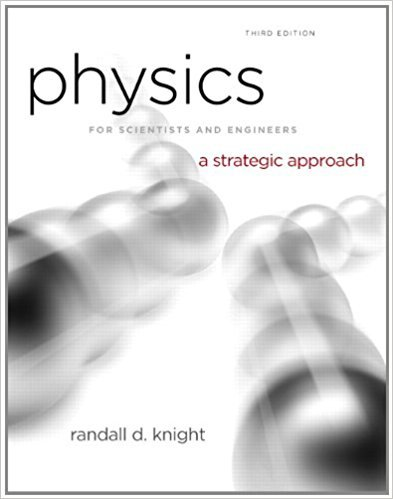×
Get Full Access to Physics For Scientists And Engineers: A Strategic Approach With Modern Physics - 3 Edition - Chapter 2 - Problem 41p
Get Full Access to Physics For Scientists And Engineers: A Strategic Approach With Modern Physics - 3 Edition - Chapter 2 - Problem 41p

×

# a. What constant acceleration, in SI units, must a carISBN: 9780321740908 69

## Solution for problem 41P Chapter 2

Physics for Scientists and Engineers: A Strategic Approach with Modern Physics | 3rd Edition

• Textbook Solutions
• 2901 Step-by-step solutions solved by professors and subject experts
• Get 24/7 help from StudySoup virtual teaching assistantsPhysics for Scientists and Engineers: A Strategic Approach with Modern Physics | 3rd Edition

4 5 1 309 Reviews
24
3
Problem 41P

Problem 41P

a. What constant acceleration, in SI units, must a car have to go from zero to 60 mph in 10 s?

b. What fraction of g is this?

c. How far has the car traveled when it reaches 60 mph? Give your answer both in SI units and in feet.

Step-by-Step Solution:

Solution

Step 1 of 3

A. To find the acceleration we use the formulawhereis the acceleration,is the velocity andis the time

From the given data. Where one mile per hour is equal tokilometer per hour and one hour is equal to.Step 2 of 3

Step 3 of 3

##### ISBN: 9780321740908

Unlock Textbook Solution